StatLect

# Estimation methods

Estimation methods are general techniques that can be used to derive estimators in a parametric estimation problem.## The general framework

Let us recall the main elements of a parametric estimation problem, which were explained in detailed in the lecture on point estimation:

• we use a sampleto make statements about the probability distribution that generated the sample;

• the sampleis regarded as the realization of a random vector;

• the unknown joint distribution function of, denoted by, is assumed to belong to a set of distribution functions, called statistical model;

• the modelis put into correspondence with a setof real vectors;is called the parameter space and its elementsare called parameters;

• we denote bythe parameter associated with the unknown data-generating distribution; if several different parameters are put into correspondence with,can be any one of them;

•is called the true parameter;

• an estimator is a predefined rule (a function) that associates a parameter estimateto eachin the support of;

• the symbolis often used to denote both the estimate and the estimator and the meaning is usually clear from the context.

The aim of an estimation method is to produce a parameter estimatethat is as close as possible to the true parameter.

## Extremum estimators

Several widely employed estimators fall within the class of extremum estimators.

An estimatoris an extremum estimator if it can be represented as the solution of a maximization problem: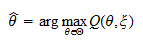whereis a function of both the parameterand the sample.

General conditions can be derived for the consistency and asymptotic normality of extremum estimators. We do not discuss them here (see, e.g., Hayashi 2000), but we give some examples of extremum estimation methods and we refer the reader to lectures that describe these examples in a more detailed manner.

## Maximum likelihood

In maximum likelihood estimation, we maximize the likelihood of the sample: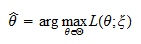where:

1. ifis discrete, the likelihoodis the joint probability mass function ofassociated to the distribution that corresponds to the parameter;

2. ifis absolutely continuous, the likelihood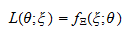is the joint probability density function ofassociated to the distribution that corresponds to the parameter.

The vectoris called the maximum likelihood estimator of.

The maximum likelihood estimation method is discussed in more detail in the lecture entitled Maximum Likelihood.

## Generalized method of moments

In the generalized method of moments (GMM) estimation method, the distribution associated to the parametersatisfies a moment condition:whereis a (vector) function andindicates that the expected value is computed using the distribution associated to.

The GMM estimatoris obtained aswhereis a measure of the distance offrom its expected value ofand the estimator is an extremum estimator because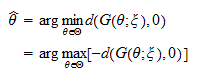## Least squares

In the least squares estimation method, the samplecomprises:

•realizationsof a random variable, called the dependent variable

•realizations, ...,of a random vector, whose components are called independent variables.

It is postulated that there exists a functionsuch that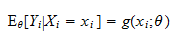The least squares estimatoris obtained as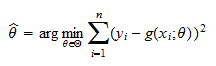The estimator is an extremum estimator because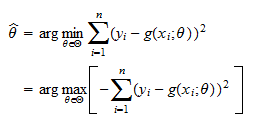A special case of the least squares estimator is analyzed in detail in the lecture on the properties of the OLS estimator.

## References

Hayashi, F. (2000) Econometrics, Princeton University Press.